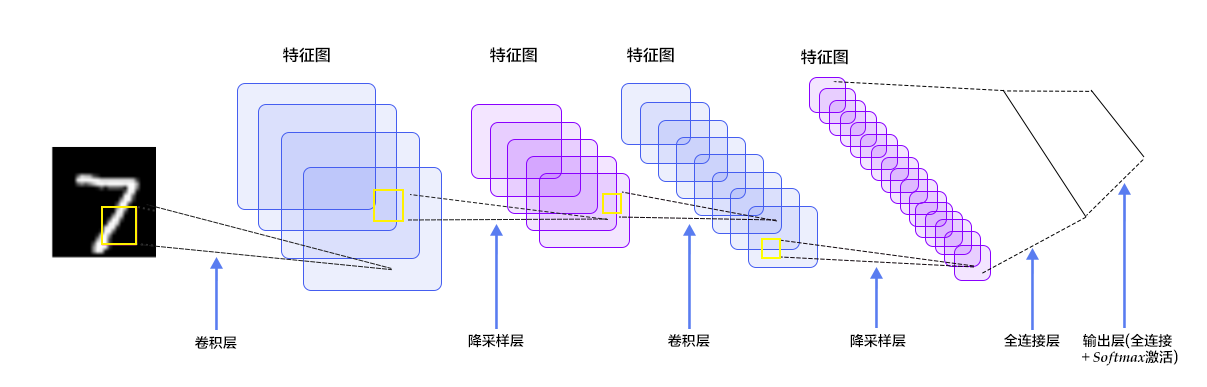## 利用飞桨实现数字识别## 概述

• X，代表输入：MNIST图片是28×28 的二维图像，为了进行计算，我们将其转化为784维向量，即X=($x_0,x_1,…,x_{783}$)。
• Y，代表分类器预测的输出：分类器的输出是10类数字（0-9），即Y=($y_0,y_1,…,y_9$)，每一维$y_i$代表图片分类为第i类数字的概率。
• Labe，代表数字图片的真实标签：Label=($l_0,l_1,…,l_9$)，也是10维，但只有一个特征为1，其他都为0，即用稀疏矩阵表示数字。例如某张图片上的数字为2，则它对应的样本为(0,0,1,0,…,0)

### Softmax回归(Softmax Regression)

X传到输出层，在激活操作之前，会乘以相应的权重 W ，并加上偏置变量 b ，如下式所示：

$$y_i = softmax(\sum_jW_{i,j}x_j + b_i)$$

$$softmax(x_i) = \frac{e^{x_i}}{\sum_je^{x_j}}$$

$$L_{cross-entropy}(label, y) = -\sum_i label_i log(y_i)$$

### 多层感知机(Multilayer Perceptron, MLP)

Softmax回归模型采用了最简单的两层神经网络，即只有输入层和输出层，因此其拟合能力有限。为了达到更好的识别效果，我们考虑在输入层和输出层中间加上若干个隐藏层。

1. 经过第一个隐藏层，可以得到 $H_1=ϕ(W_1X+b_1)$，其中ϕ代表激活函数，常见的有sigmoid、tanh或ReLU等函数。
2. 经过第二个隐藏层，可以得到 $H_2=ϕ(W_2H_1+b_2)$。
3. 最后，再经过输出层，得到的$Y=softmax(W_3H_2+b_3)$，即为最后的分类结果。

### 卷积神经网络(Convolutional Neural Network, CNN)## 操作过程

PaddlePaddle在API中提供了自动加载MNIST数据的模块paddle.dataset.mnist。加载后的数据位于/home/username/.cache/paddle/dataset/mnist下：

train-images-idx3-ubyte 训练数据图片，60,000条数据
train-labels-idx1-ubyte 训练数据标签，60,000条数据
t10k-images-idx3-ubyte 测试数据图片，10,000条数据
t10k-labels-idx1-ubyte 测试数据标签，10,000条数据

### Fluid API 概述

1. inference_program：指定如何从数据输入中获得预测的函数， 这是指定网络流的地方。
2. train_program：指定如何从 inference_program 和标签值中获取 loss 的函数， 这是指定损失计算的地方。
3. optimizer_func: 指定优化器配置的函数，优化器负责减少损失并驱动训练，Paddle 支持多种不同的优化器。

#### Program Functions 配置

• Softmax回归：只通过一层简单的以softmax为激活函数的全连接层，就可以得到分类的结果。
• 多层感知器：下面代码实现了一个含有两个隐藏层（即全连接层）的多层感知器。其中两个隐藏层的激活函数均采用ReLU，输出层的激活函数用Softmax。
• 卷积神经网络LeNet-5: 输入的二维图像，首先经过两次卷积层到池化层，再经过全连接层，最后使用以softmax为激活函数的全连接层作为输出层。

#### 构建训练过程

##### Event Handler 配置

event_handler 用来在训练过程中输出训练结果

event_handler_plot 可以用来在训练过程中画图如下：### 应用模型

#### 生成预测输入数据

infer_3.png 是数字 3 的一个示例图像。把它变成一个 numpy 数组以匹配数据feed格式。

#### Inference 创建及预测